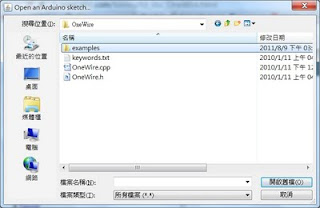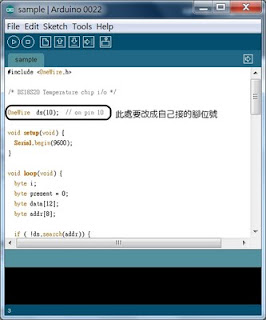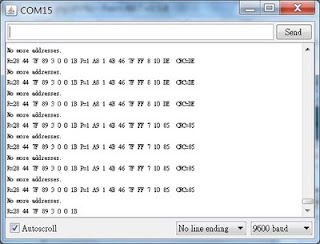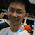2011年8月10日 星期三

Arduino 溫度感應DS18B20防水感應器電阻板DS18B20 接法麵包板接 DS18B20OneWire example修改程式腳位DS18B20 傳回 0x28Serial Monitor

First off, you need to define some variables, (put right under loop() above)int HighByte, LowByte, TReading, SignBit, Tc_100, Whole, Fract; Then for a DS18B20 series you will add the following code below the
`Serial.println();` above LowByte = data; HighByte = data; TReading = (HighByte << 8) + LowByte; SignBit = TReading & 0x8000; // test most sig bit if (SignBit) // negative { TReading = (TReading ^ 0xffff) + 1; // 2's comp } Tc_100 = (6 * TReading) + TReading / 4; // multiply by (100 * 0.0625) or 6.25 Whole = Tc_100 / 100; // separate off the whole and fractional portions Fract = Tc_100 % 100; if (SignBit) // If its negative { Serial.print("-"); } Serial.print(Whole); Serial.print("."); if (Fract < 10) { Serial.print("0"); } Serial.print(Fract); Serial.print("\n");Serial Monitor 傳回溫度

1 則留言:

1.hello你好
我們新竹電料行可以單買電阻耶
一個6毛

這篇文章很有參考價值~~~
推~